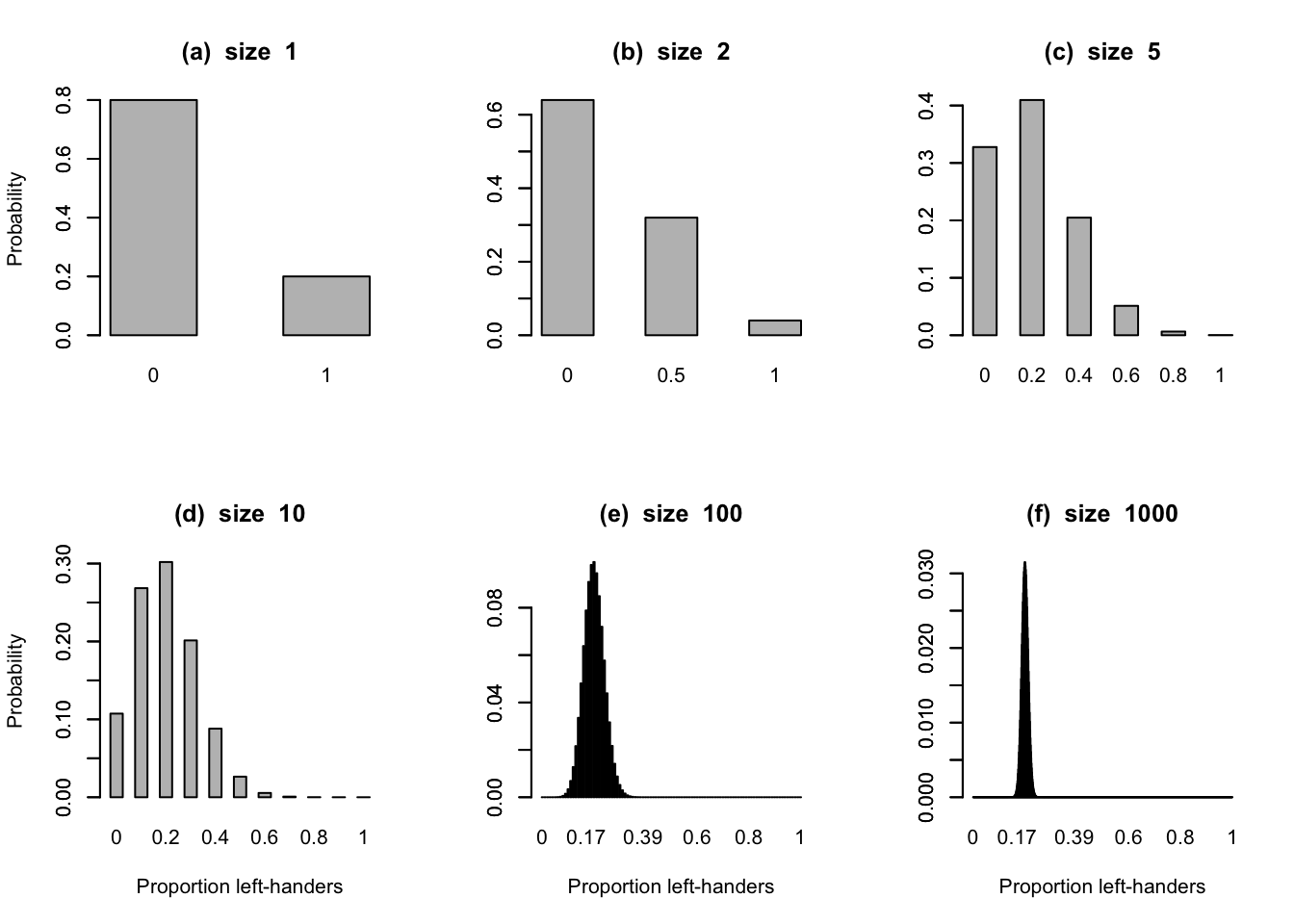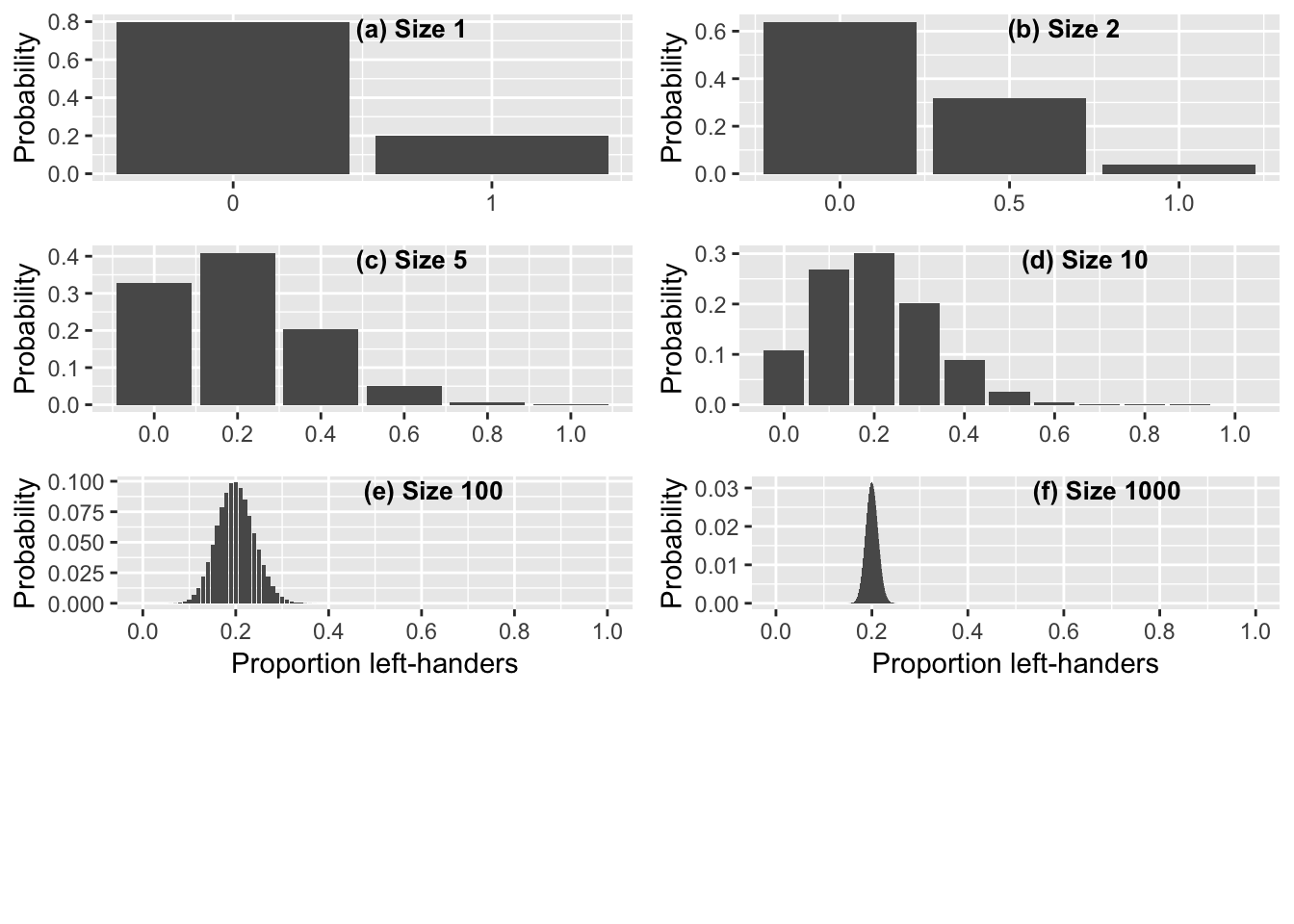First in R base graphics

#9-1
library(magrittr)
library(ggplot2)
library(ggpubr)
library(gridExtra)

n<-c(1,2,5,10,100,1000)
pleft=0.2
scale<-1.0
lets<-c("(a)","(b)","(c)","(d)","(e)","(f)")
mains<-paste(lets, " size ",n)
xlabs = c("","","","Proportion left-handers","Proportion left-handers","Proportion left-handers")
ylabs = c("Probability","","","Probability","","","")

par(mfrow=c(2,3))
scale=0.9
for(i in 1:6){
x<-seq(0,n[i])
y<-dbinom(x,n[i],pleft)
barplot(y,space=1, names.arg=x/n[i], xlab=xlabs[i],ylab=ylabs[i],main=mains[i])
axis(2,cex=scale)
}Figure 9.1 The probability distribution of the observed proportion of left-handers in random samples of 1, 2, 5, 10, 100 and 1,000 people, where the true underlying proportion of left-handers in the population is 0.2. The probability of getting at least 30% left-handers in the sample is found by adding all the probability in the bars to the right of 0.3.

Now in ggplot

P <- list() # prepare list for plots
for(i in 1:6) # 6 runs with parameter i

#Since for-loops have no separate variable scope (i.e. they are performed in the current environment) we need to use local to wrap the for block

local({
i <- i #in addition, we need to make i a local variable -which we can do by simply re-assigning it

x <-seq(0,n[i])
y <-dbinom(x,n[i],pleft)
df <- cbind.data.frame(x/n[i],y)
p <- ggplot(df, aes(x=x/n[i], y=y)) # define plot object from dataframe
p <- p + geom_bar(stat="identity") # define bar-chart type

ifelse(i==1,
p <- p + scale_x_continuous(breaks=c(0, 1)),
ifelse(i==2,
p <- p + scale_x_continuous(breaks=c(0, 0.5, 1.0))
, p <- p + scale_x_continuous(breaks=c(0, 0.2, 0.4, 0.6, 0.8, 1.0))
)) # defines x-axis labels according to the number od bars and their positions

ifelse(i<5,
p <- p + labs(x=NULL, y="Probability")
,
p <- p + labs(x="Proportion left-handers", y="Probability") # x-axis label only required on bottom two ggplots
)

P[[i]] <<- p # add each plot into plot list

})

g <- ggarrange(P[],P[],P[],P[],P[],P[], ncol=2 , nrow=4,labels=c("(a) Size 1", "(b) Size 2", "(c) Size 5", "(d) Size 10", "(e) Size 100", "(f) Size 1000"), font.label = list(size = 10, color = "black"), hjust = c(-3.2,-3.2,-3.2,-2.95,-2.6,-2.6), vjust = 2) # display the plots, NOTE that [[]]'s are required to access the list objects as ggplots

# include to produce ps version of graph
#setEPS()
#postscript("09-1-left-hander-binomial.eps")

g # displays the ggarranged ggplots# include to produce ps version of graph
#dev.off()

Probabilities of getting at least 30%

pbinom(0.3*n,n,pleft,lower.tail=F)
##  2.000000e-01 3.600000e-01 2.627200e-01 1.208739e-01 6.059335e-03
##  2.201699e-14